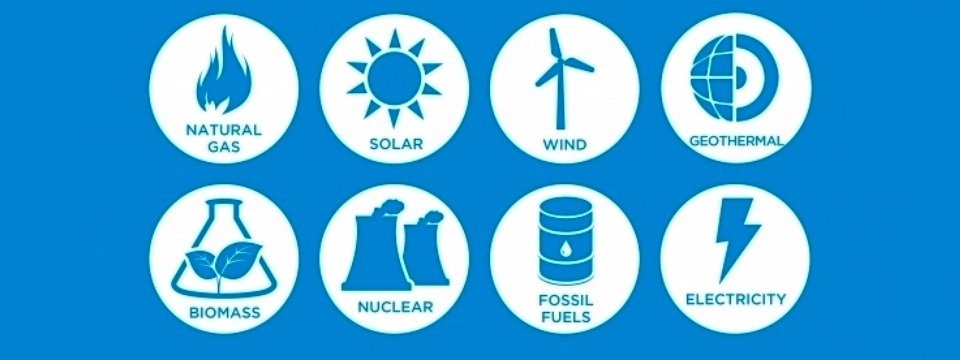Posted by on Sep 18, 2017 in Tell Me Why Numerous Questions and Answers |# What Is Energy?

Energy is the power to act. There are several different forms of energy, and one kind can be changed into another kind. But although it can be changed from one form into another it can never be lost. The total amount of energy in the universe remains constant.

If you strike a match on the side of a match-box, mechanical and chemical energy is changed into heat energy. If you use a saw, the blade gets hot because the energy of movement or mechanical energy becomes heat energy. The end of a bicycle pump becomes warm after pumping because heat is generated when air is compressed.

In a simple electric circuit there are several changes. The battery has chemical energy, which is converted to electrical energy, which produces light and heat energy in the bulb. We depend for a large part of our power on steam, diesel and petrol engines in which the chemical energy stored in a fuel is converted first into heat energy, which is then turned into mechanical energy.

In physics, energy is the property that must be transferred to an object in order to perform work on, or to heat, the object. It can be converted in form, but not created or destroyed. The SI unit of energy is the joule, which is the energy transferred to an object by the work of moving it a distance of 1 metre against a force of 1 newton.

Common forms of energy include the kinetic energy of a moving object, the potential energy stored by an object’s position in a force field (gravitational, electric or magnetic), the elastic energy stored by stretching solid objects, the chemical energy released when a fuel burns, the radiant energy carried by light, and the thermal energy due to an object’s temperature.

Mass and energy are closely related. Due to mass–energy equivalence, any object that has mass when stationary (called rest mass) also has an equivalent amount of energy whose form is called rest energy (in that frame of reference), and any additional energy (of any form) acquired by the object above that rest energy will increase the object’s total mass just as it increases its total energy. For example, after heating an object, its increase in energy could be measured as an increase in mass, with a sensitive enough scale.Living organisms require available energy to stay alive, such as the energy humans get from food. Humans get the energy they need from energy resources such as fossil fuels, nuclear fuel, or renewable energy. The processes of Earth’s climate and ecosystem are driven by the radiant energy Earth receives from the sun and the geothermal energy contained within the earth.

The total energy of a system can be subdivided and classified in various ways. For example, classical mechanics distinguishes between kinetic energy, which is determined by an object’s movement through space, and potential energy, which is a function of the position of an object within a field.

It may also be convenient to distinguish gravitational energy, thermal energy, several types of nuclear energy (which utilize potentials from the nuclear force and the weak force), electric energy (from the electric field), and magnetic energy (from the magnetic field), among others. Many of these classifications overlap; for instance, thermal energy usually consists partly of kinetic and partly of potential energy.Some types of energy are a varying mix of both potential and kinetic energy. An example is mechanical energy which is the sum of (usually macroscopic) kinetic and potential energy in a system. Elastic energy in materials is also dependent upon electrical potential energy (among atoms and molecules), as is chemical energy, which is stored and released from a reservoir of electrical potential energy between electrons, and the molecules or atomic nuclei that attract them.

The list is also not necessarily complete. Whenever physical scientists discover that a certain phenomenon appears to violate the law of energy conservation, new forms are typically added that account for the discrepancy.

Heat and work are special cases in that they are not properties of systems, but are instead properties of processes that transfer energy. In general we cannot measure how much heat or work are present in an object, but rather only how much energy is transferred among objects in certain ways during the occurrence of a given process. Heat and work are measured as positive or negative depending on which side of the transfer we view them from.

Potential energies are often measured as positive or negative depending on whether they are greater or less than the energy of a specified base state or configuration such as two interacting bodies being infinitely far apart. Wave energies (such as radiant or sound energy), kinetic energy, and rest energy are each greater than or equal to zero because they are measured in comparison to a base state of zero energy: “no wave”, “no motion”, and “no inertia”, respectively.

The distinction between different kinds of energy is not always clear-cut. As Richard Feynman points out:

These notions of potential and kinetic energy depend on a notion of length scale. For example, one can speak of macroscopicpotential and kinetic energy, which do not include thermal potential and kinetic energy. Also what is called chemical potential energy is a macroscopic notion, and closer examination shows that it is really the sum of the potential and kinetic energy on the atomic and subatomic scale.

Similar remarks apply to nuclear “potential” energy and most other forms of energy. This dependence on length scale is non-problematic if the various length scales are decoupled, as is often the case … but confusion can arise when different length scales are coupled, for instance when friction converts macroscopic work into microscopic thermal energy.Content for this question contributed by Kathy Misuir, resident of Roselle Park, Union County, New Jersey, USA